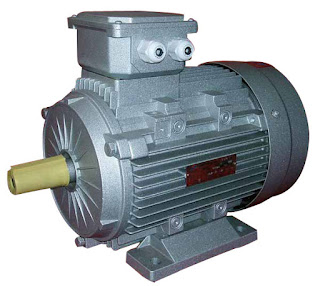General Curve Using the  induction machine power and torque  equations it is possible to produce the torque speed curve shown below....

### General Curve

Using the induction machine power and torque equations it is possible to produce the torque speed curve shown below.The torque speed curve is approximately symmetric about synchronous speed. (The magnitude of the peak negative torque is larger than the positive torque, the difference being a function of stator resistance)

#### Operating Regions

The torque-speed curve brakes down into three operating regions:
1. Braking, ${n}_{m}<0$$s>1$
Torque is positive whilst speed is negative. Considering the power conversion equation
${P}_{conv}=\left(1-s\right){P}_{gap}$
it can be seen that if the power converted is negative (from $P=\tau \omega$ ) then the airgap power is positive. i.e. the power is flowing from the stator to the rotor and also into the rotor from the mechanical system. This operations is also called plugging.
This mode of operation can be used to quickly stop a machine. If a motor is travelling forwards it can be stopped by interchanging the connections to two of the three phases. Switching two phases has the result of changing the direction of motion of the stator magnetic field, effectively putting the machine into braking mode in the opposite direction.
2. Motoring, $0<{n}_{m}<{n}_{s}$$0
Torque and motion are in the same direction. This is the most common mode of operation.
3. Generating,${n}_{m}>{n}_{s}$$s<0$
In this mode, again torque is positive whilst speed is negative. However, unlike plugging,
${P}_{conv}=\left(1-s\right){P}_{gap}$
indicates that if the power converted is negative, so is the air gap power. In this case, power flows from the mechanical system, to the rotor circuit, then across the air gap to the stator circuit and external electrical system.

## The torque equation

Using the equation
$\tau =\frac{3{I}_{2}^{2}{R}_{2}}{s{\omega }_{s}}$
the torque speed curve can be solved by first solving the circuit model to find the rotor current. In the absence of a computer, this is a tedious process at best. Multiple solutions of the above equation for torque at different slips can be made simpler by simplifying the equivalent circuit model. Consider the diagram below:The stator part of the equivalent circuit (together with the magnetising branch) can be replaced by a Thevenin equivalent circuit. In the Thevenin circuit, the stator phase voltage has been replaced by its Thevenin equivalent,
$\begin{array}{rl}{\stackrel{\to }{V}}_{TH}& =\frac{j{X}_{m}}{{R}_{1}+j\left({X}_{1}+{X}_{m}\right)}{\stackrel{\to }{V}}_{1}\\ {V}_{TH}& =|{\stackrel{\to }{V}}_{TH}|\end{array}$
and the impedances have been replaced by Thevenin equivalent impedances.
$\begin{array}{rl}{Z}_{TH}& =\left({R}_{1}+j{X}_{1}\right)||j{X}_{m}\\ {Z}_{TH}& =\frac{j{X}_{m}\left({R}_{1}+j{X}_{1}\right)}{{R}_{1}+j{X}_{1}+j{X}_{m}}\\ {Z}_{TH}& ={R}_{TH}+j{X}_{TH}\end{array}$
Incorporating the Thevenin model into the circuit model results in the Thevenin equivalent circuit model of an induction machine.In the above circuit, the calculation of rotor current is greatly simplified
$\begin{array}{rl}{\stackrel{\to }{I}}_{2}& =\frac{{\stackrel{\to }{V}}_{TH}}{{Z}_{TH}+{Z}_{2}}\\ {\stackrel{\to }{I}}_{2}& =\frac{{\stackrel{\to }{V}}_{TH}}{\left({R}_{TH}+\frac{{R}_{2}}{s}\right)+j\left({X}_{TH}+{X}_{2}\right)}\end{array}$
The above expression for rotor current can be squared and substituted into the torque equation
$\begin{array}{rl}{\stackrel{\to }{I}}_{2}^{2}& =\frac{{V}_{TH}^{2}}{{\left({R}_{TH}+\frac{{R}_{2}}{s}\right)}^{2}+{\left({X}_{TH}+{X}_{2}\right)}^{2}}\\ \tau & =\frac{3{V}_{TH}^{2}}{{\left({R}_{TH}+\frac{{R}_{2}}{s}\right)}^{2}+{\left({X}_{TH}+{X}_{2}\right)}^{2}}\frac{{R}_{2}}{s{\omega }_{s}}\end{array}$
Using the above equation, the variation of torque with slip can be plotted directly .
Note that if power or efficiency calculations are needed, the full equivalent circuit model should be used (not the Thevenin version).

## Motoring Torque Characteristic

The motoring region of the induction machine torque-speed curve is the region of greatest interest. The plots below show a number of different torque speed curves, due to differences in the motor designs. Common features of interest are noted and discussed below.• τrated . The rated torque of the machine. This is the design operating point.
• τstart . The start torque of the machine, when the machine is at standstill.
• τmax or τpo . The maximum torque or pull-out torque. Once a machine has reached rated operating point, this is the maximum torque that can be applied without stopping the machine (pulling out). In reality, since this torque is significantly more than the design rated torque, operation at maximum torque is not possible due to thermal heating issues. (Currents will be above rated values, I2R losses will be excessive.)
• τpu . The pull-up torque of the machine. In some machines, the lowest point on the torque speed curve between starting and pullout is not the start torque. In this case it is important to know the pull-up torque. This is the minimum torque that the motor can accelerate up to the desired operating speed.
In steady state operation, an induction motor will operate at the speed where the mechanical load torque equals the torque developed by the motor. At low speeds, the difference between the motor torque and the load torque accelerates the machine. Normal operation is to the right of the maximum torque. In this region, an increase in the load torque will cause the motor to slow, increasing the motor torque until an equilibrium is reached. To the left of the pullout torque, no such equilibrium can be reached. Mechanically,
${\tau }_{motor}-{\tau }_{load}=J\frac{d{\omega }_{m}}{dt}$
where J is the rotational inertia of the mechanical system.Name

BASIC ELECTRICAL,12,BATTERIES,4,CIRCUIT THEORIES,9,CONTROL SYSTEMS,3,DC MOTOR,1,DIGITAL ELECTRONICS,1,DISTRIBUTED GENERATION,2,DISTRIBUTION,6,ELECTRICAL DRIVES,1,ELECTRICAL LAWS,8,ELECTRONICS DEVICES,2,General,7,GENERATION,3,GENERATOR,1,HIGH VOLTAGE,4,ILLUMINATION,1,INDUCTION MOTOR,7,MATERIALS,1,MEASUREMENT,1,MOTOR,1,POWER ELECTRONICS,2,PROJECTS ON INDUCTION MOTOR,1,PROTECTION,1,SMART GRID,3,SWITCHGEAR,4,SYNCHRONOUS MOTOR,1,TRANSFORMER,6,TRANSMISSION,4,
ltr
item
Electrical for Us: Inducton Machine Torque Speed Curve
Inducton Machine Torque Speed Curve
https://2.bp.blogspot.com/-bq6tkQcOFWM/WEppKW_9XGI/AAAAAAAAAgs/HsrrlTyegh0a1W8gC5XlYZxWpTRHOzORwCEw/s320/20110305120000000859451.jpg
https://2.bp.blogspot.com/-bq6tkQcOFWM/WEppKW_9XGI/AAAAAAAAAgs/HsrrlTyegh0a1W8gC5XlYZxWpTRHOzORwCEw/s72-c/20110305120000000859451.jpg
Electrical for Us
http://www.sanjaysah.com.np/2016/12/inducton-machine-torque-speed-curve.html
http://www.sanjaysah.com.np/
http://www.sanjaysah.com.np/
http://www.sanjaysah.com.np/2016/12/inducton-machine-torque-speed-curve.html
true
3851448774078769448
UTF-8